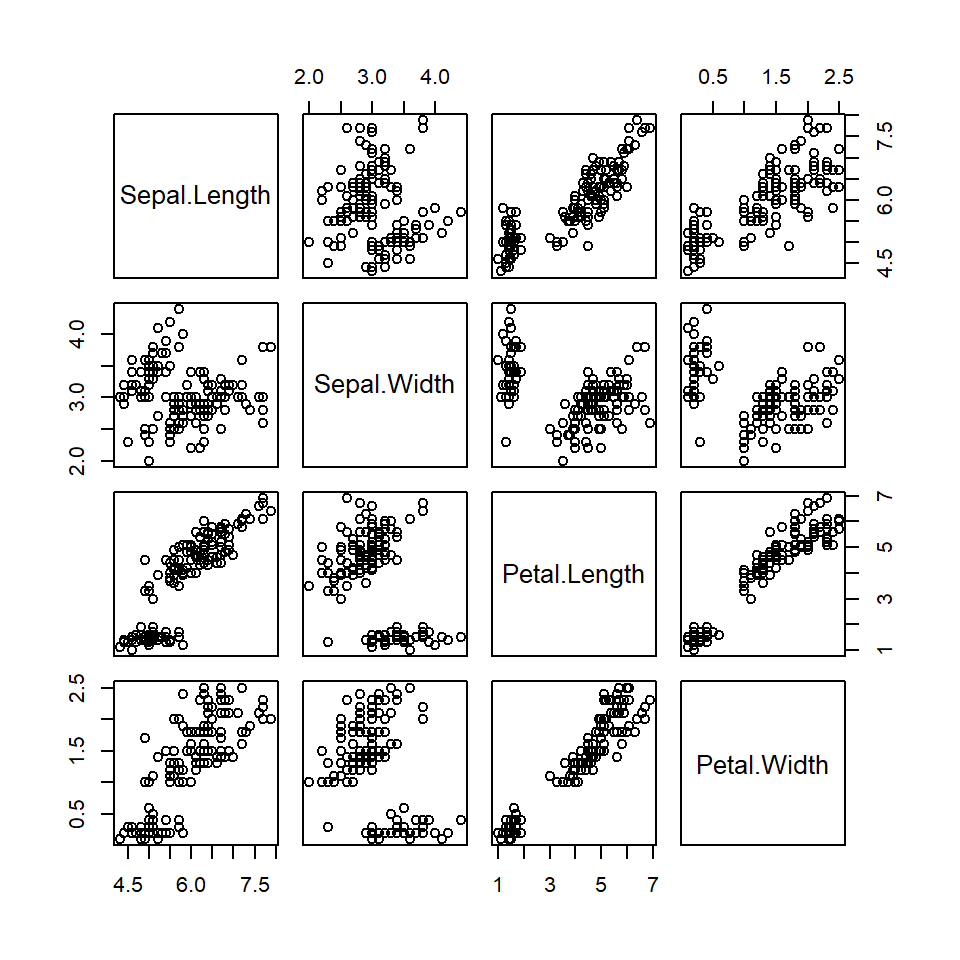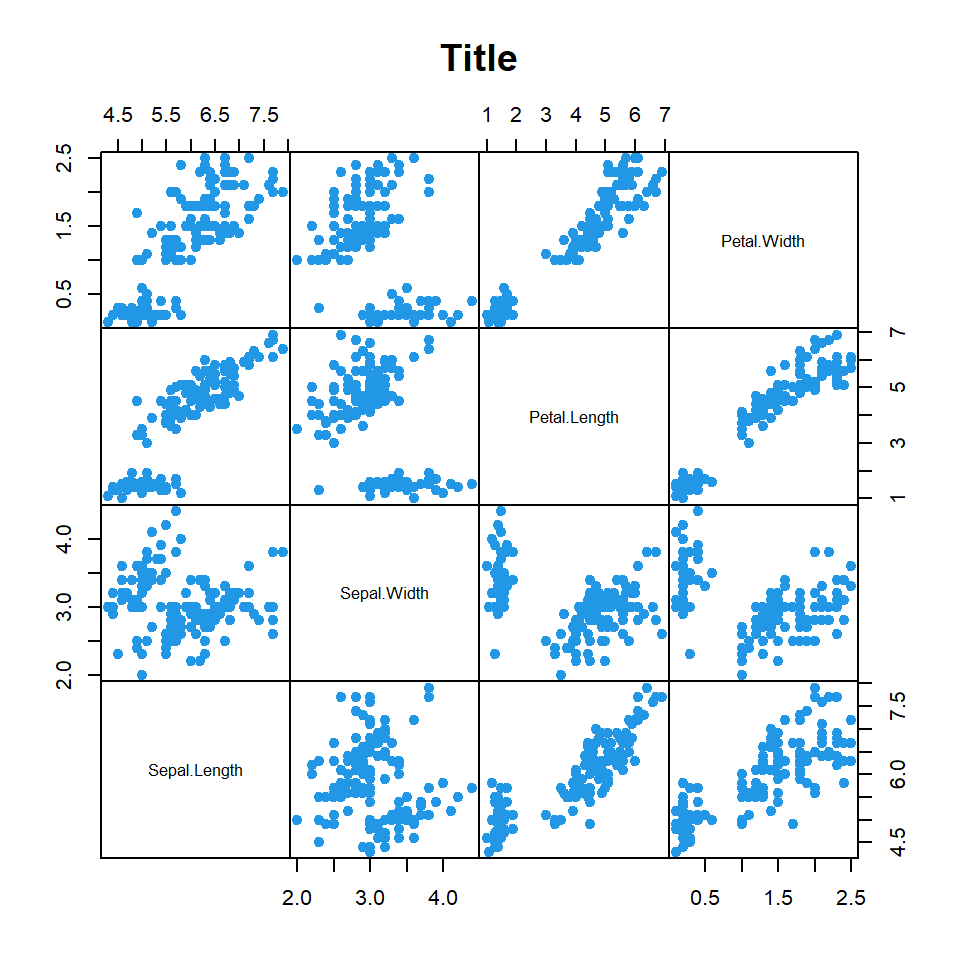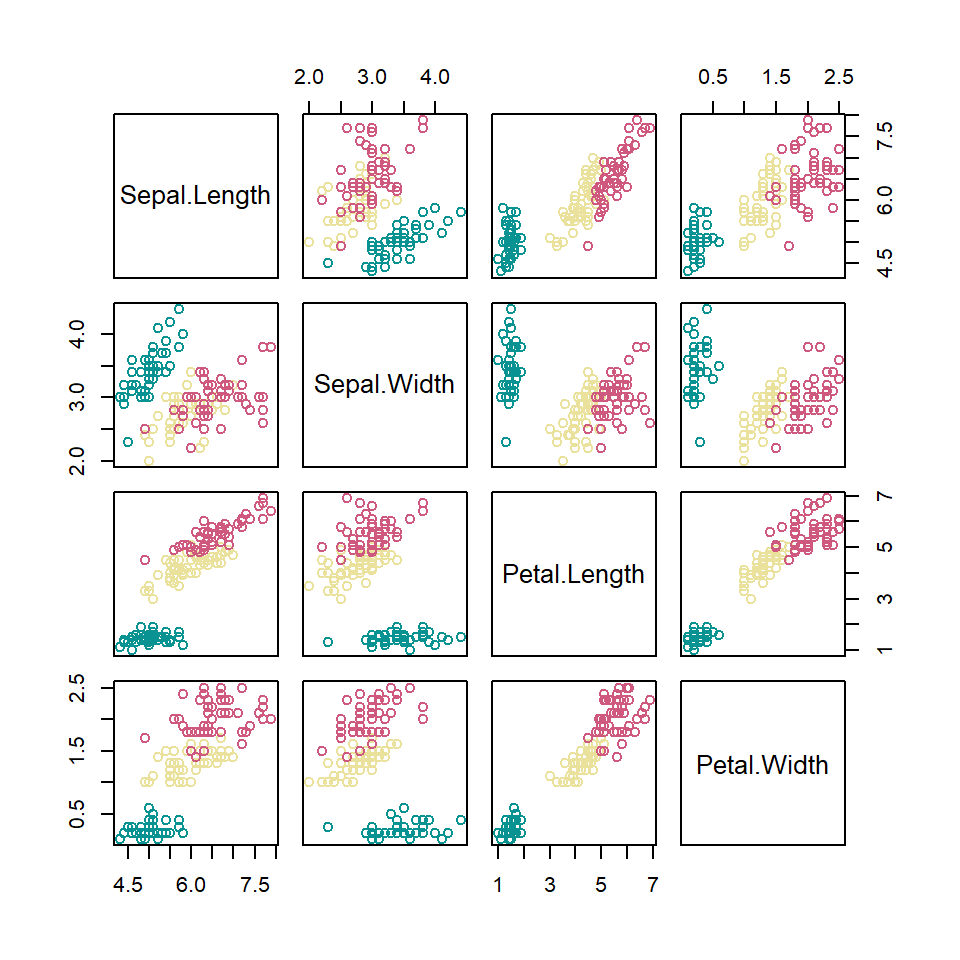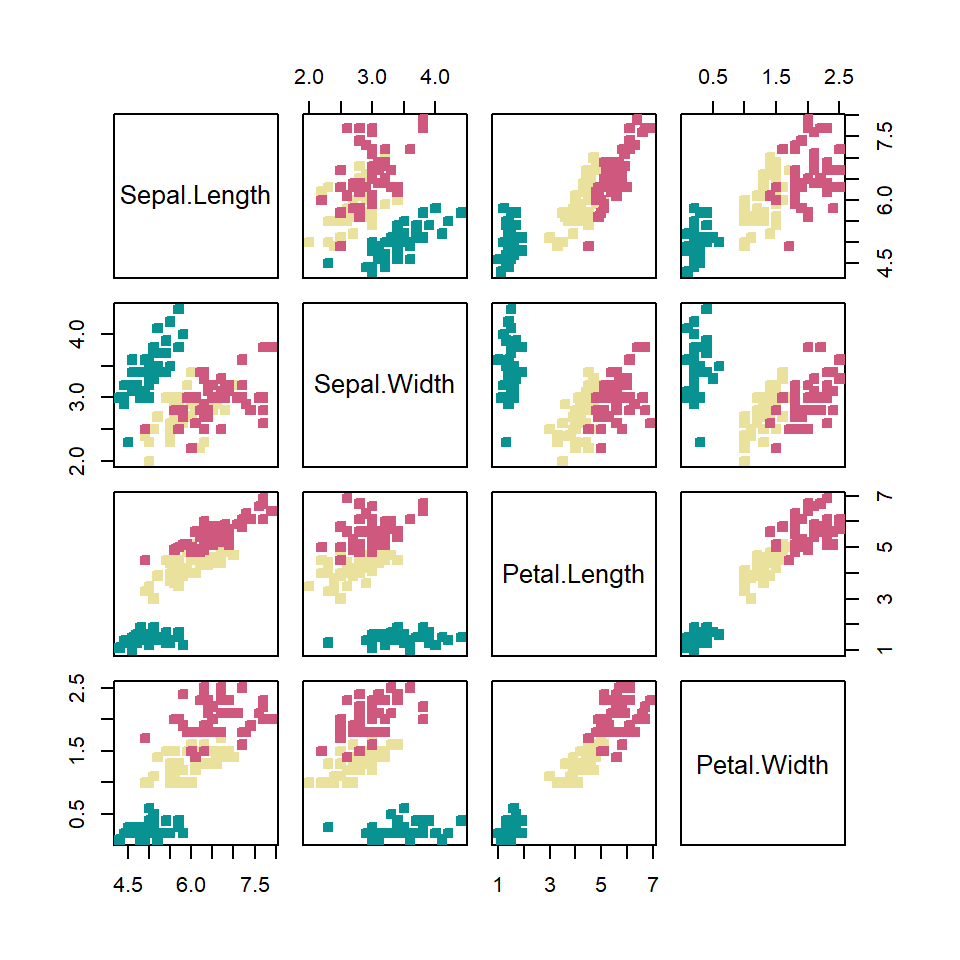# pairs function in R

## The `pairs` function

In base R you can create a pairwise correlation plot with the `pairs` function. Note that this is the same as plotting a numeric data frame with `plot`.

``````# Numeric variables
df <- iris[1:4]

pairs(df)

# Equivalent to:
pairs(~ Sepal.Length + Sepal.Width +
Petal.Length + Petal.Width, data = df)

# Equivalent to:
with(df, pairs(~ Sepal.Length + Sepal.Width +
Petal.Length + Petal.Width))

# Equivalent to:
plot(df)``````There are several arguments that can be used to customize the resulting output. The following block of code highlights and describes some of the most relevant arguments. Type `?pairs` for the full list.

``````# Numeric variables
df <- iris[1:4]

pairs(df,       # Data
pch = 19, # Pch symbol
col = 4,  # Color
main = "Title",    # Title
gap = 0,           # Subplots distance
row1attop = FALSE, # Diagonal direction
labels = colnames(df), # Labels
cex.labels = 0.8,  # Size of diagonal texts
font.labels = 1)   # Font style of diagonal texts``````## Color by groupThe color scheme can be customized so each group of your data set can be colorized on a different color. For that purpose you can index your grouping variable to a color palette. Check the list of color palettes or create your own.

``````# Numeric variables
df <- iris[1:4]

# Groups
species <- iris[, 5]

# Number of groups
l <- length(unique(species))

pairs(df, col = hcl.colors(l, "Temps")[species])``````Note that some pch symbols allow you to set a background and a border color as in the following example.

``````# Numeric variables
df <- iris[1:4]

# Groups
species <- iris[, 5]

# Number of groups
l <- length(unique(species))

pairs(df,
pch = 22,
bg = hcl.colors(l, "Temps")[species],
col = hcl.colors(l, "Temps")[species])``````